## Склад секції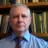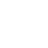Alexander L. Yampolsky Docent (associate professor) of department of fundamental mathematics, z, doctor of sciences in physics and mathematics, docent (associate professor)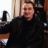Dmytro V. Bolotov Doctor of sciences in physics and mathematics, senior researcher of iltpe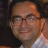Vasyl O. Gorkavyy Doctor of sciences in physics and mathematics, docent (associate professor)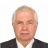. Docent (associate professor) of department of theoretical and applied computer science , phd in mathematics, docent (associate professor)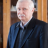P. G. Dolya Docent (associate professor) of department of theoretical and applied computer science , phd for industries

## Schedule for today

 O.O. Shugailo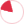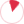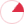Week schedule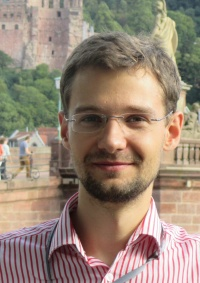# Kostiantyn D. Drach

Phd in mathematics

## List of selected publications

K. Drach Some sharp estimates for convex hypersurfaces of pinched normal curvature // Journal of Mathematical Physics, Analysis, Geometry, vol. 11, No. 2, pp. 111-122, 2015

For a convex domain $D$ bounded by the hypersurface $\partial D$ in a space of constant curvature we give sharp bounds on the width $R-r$ of a spherical shell with radii $R$ and $r$ that can enclose $\partial D$, provided that normal curvatures of $\partial D$ are pinched by two positive constants. Furthermore, in the Euclidean case we also present sharp estimates for the quotient $R/r$. From the obtained estimates we derive stability results for almost umbilical hypersurfaces in the constant curvature spaces.

Keywords: convex hypersurface, spaces of constant curvature, pinched normal curvature, $\lambda$-convexity, spherical shell

A.A. Borisenko, K.D. Drach Comparison theorem for support functions of hypersurfaces // Reports of the National Academy of Sciences of Ukraine, (3): 11-16, 2015

For a convex domain $D$ that is enclosed by the hypersurface $\partial D$ of bounded normal curvature, we prove an angle comparison theorem for angles between $\partial D$ and geodesic rays starting from some fixed point in $D$, and the corresponding angles for hypersurfaces of constant normal curvature. Also, we obtain a comparison theorem for support functions of such surfaces. As a corollary, we present a proof of Blaschke's Rolling Theorem.

Keywords: comparison theorems, normal curvature, Blaschke's Rolling Theorem, Riemannian support function, polar map

K. Drach About an isoperimetric property of $\lambda$-convex lunes on the Lobachevsky plane // Reports of the National Academy of Sciences of Ukraine, (11): 11-15, 2014

We give a sharp lower bound on the area of a domain that can be enclosed by a closed embedded $\lambda$-convex curve of a given length on the Lobachevsky plane.

Keywords: $\lambda$-convex curves, reverse isoperimetric inequality, Pontryagin's Maximum Principle

A.A. Borisenko, K.D. Drach Isoperimetric Inequality for Curves with Curvature Bounded Below // Mathematical Notes, Vol. 95, No. 5, pp. 14–22., 2014

For embedded closed curves with curvature bounded below, we prove an isoperimetric inequality estimating the minimal area bounded by such curves for a ﬁxed perimeter.

Keywords: isoperimetric inequality, embedded closed curve, minimal area

A.A. Borisenko, K.D. Drach Extreme properties of curves with bounded curvature on a sphere // Journal of Dynamical and Control Systems, (DOI) 10.1007/s10883-014-9221-z, 2014

We give a sharp lower bound on the area of the domain enclosed by an embedded curve lying on a two-dimensional sphere, provided that geodesic curvature of this curve is bounded from below. Furthermore, we prove some dual inequalities for convex curves whose curvatures are bounded from above.

Keywords: $\lambda$-convex curves \and reverse isoperimetric inequality \and Pontryagin's Maximum Principle

A.A. Borisenko, K.D. Drach Closeness to spheres of hypersurfaces with normal curvature bounded below // Sbornik: Mathematics, 204 (11): 1565-1583, 2013

For a Riemannian manifold $M^{n+1}$ and a compact domain $\Omega \subset M^{n+1}$ bounded by a hypersurface $\partial \Omega$ with normal curvature bounded below, estimates are obtained in terms of the distance from $O$ to $\partial \Omega$ for the angle between the geodesic line joining a fixed interior point $O$ in $\Omega$ to a point on $\partial \Omega$ and the outward normal to the surface. Estimates for the width of a spherical shell containing such a hypersurface are also presented.

Keywords: Riemannian manifold, sectional curvature, normal curvature of a hypersurface, comparison theorems, $\lambda$-convex hypersurface

A.A. Borisenko, K.D. Drach About an angle comparison theorem for closed curves // Reports of NAS of Ukraine, (6): 7-11, 2011

We estimate an angle between the radius vector from the inner point of the closed regular curve and its outer normal depending on the distance between the point and the curve. The cases of the complete connected two-dimensional manifold with constant and non-constant curvature were considered.

K. Drach Bolyai-Gerwien theorem for unbounded polygons // Preprint, 2015

Using the notion of a limiting angle we prove a version of the classical Bolyai - Gerwien theorem for unbounded polygons on the Euclidean plane.

K. Drach, M. Mixer Minimal covers of equivelar toroidal maps // Ars Mathematica Contemporanea, 9:2, 77-91, 2015

Given any equivelar map on the torus, it is natural to consider its covering maps. The most basic of these coverings are finite toroidal maps or infinite tessellations the Euclidean plane. In this paper, we prove that each equivelar map on the torus has a unique minimal toroidal rotary cover and also a unique minimal toroidal regular cover. That is to say, of all the toroidal rotary (or regular) maps covering a given map, there is a unique smallest. Furthermore, using the Gaussian and Eisenstein integers, we construct these covers explicitly.

Keywords: Minimal covers, Regular and rotary maps, Gaussian and Eisenstein integers

K. Drach, Yu. Eremenko, A. Krymova Uniquely constructed figures on the sphere // Preprint (ukr), 2014

On the plane two figures are equidecomposable if we can cut one of them with straight cuts into finitely many pieces, and rearrange them into the second one. If there is a convex figure which is not equidecomposable to any convex figure we call it uniquely constructed figure. In this work we study uniquely constructed convex figures on the unit sphere. As the main result of this research, we prove the following theorem: on a sphere a lens, that is an intersection of two discs with the same radius, is a uniquely constructed figure. Since a circle is the special case of a lens, from the previous theorem we get the circle on a sphere is a uniquely constructed figure.